### DEGREES Function – Radians to Degrees – Excel, VBA & G Sheets

This Tutorial demonstrates how to use the Excel DEGREES Function in Excel to convert radians to degrees.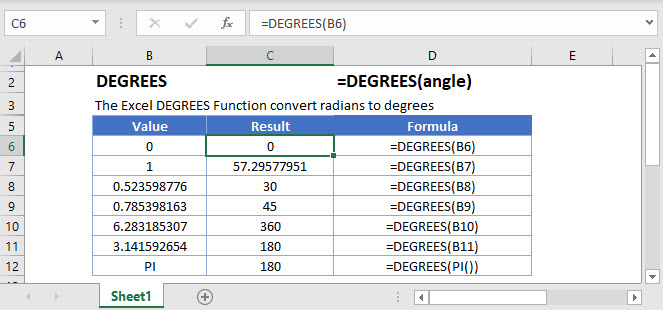## DEGREES Function Overview

The DEGREES Function Convert radians to degrees.

To use the DEGREES Excel Worksheet Function, select a cell and type: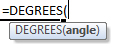(Notice how the formula inputs appear)

## DEGREES function Syntax and inputs:

angle – Angle in radians that you want to convert to degrees.

AutoMacro - VBA Code Generator

## DEGREES Function

The DEGREES Function converts radians into degrees.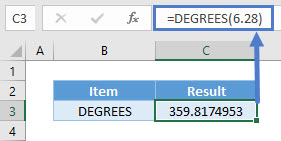## DEGREES & PI Function

The DEGREES Function will return 180 degrees when the PI Function is entered as an argument.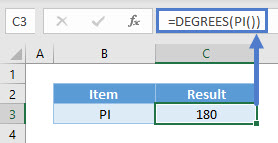## DEGREES Function – Negative Angle

The DEGREES Function can also convert negative angles into degrees.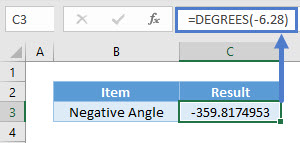## DEGREES in Google Sheets

The DEGREES Function works exactly the same in Google Sheets as in Excel: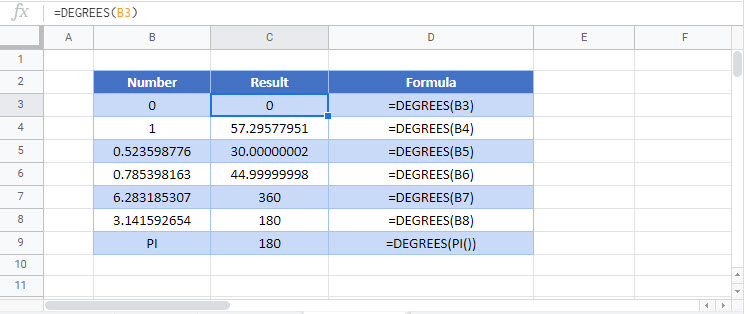## Additional Notes

Use the DEGREES Function to convert radians to degrees. Simply enter the radians directly into the formula, or reference a cell containing the radians.

## DEGREES Examples in VBA

You can also use the DEGREES function in VBA. Type:
`application.worksheetfunction.degrees(angle)`
For the function arguments (angle, etc.), you can either enter them directly into the function, or define variables to use instead.

Return to the List of all Functions in Excel

## Excel Practice Worksheet

Practice Excel functions and formulas with our 100% free practice worksheets!

• Automatically Graded Exercises
• Learn Excel, Inside Excel!